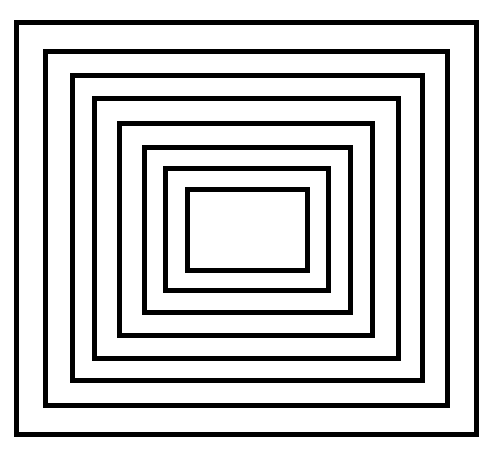QUESTION

# The adjoining figure shows a set of concentric squares. If the diagonal of the innermost square is 2 units, and if the distance between the corresponding corners of any two successive squares is 1 unit, find the difference between the values of the areas of the eight and the seventh squares, counting from the innermost square in square units.Hint: First of all, find the length of the diagonal of seventh square as the length of diagonal of seventh square is equal to sum of lengths of diagonal of the first square and twice the sum of distance between the seventh and first square at one end. Then find the area of the seventh square. Similarly find for the eighth square. And then subtract the area of seventh square from eighth to get the required answer.

So, diagonal of 7th square $= 2 + 2\left( {6 \times 1} \right) = 14$
And diagonal of 8th square $= 2 + 2\left( {7 \times 1} \right) = 16$
We know that the area of the square with diagonal $D$ is given by $\dfrac{{{D^2}}}{2}$.
So, the area of 7th square $= \dfrac{{{{\left( {14} \right)}^2}}}{2} = 98$
And the area of 8th square $= \dfrac{{{{\left( {16} \right)}^2}}}{2} = 128$
Difference of area of 8th square and 7th square $= 128 - 98 = 30{\text{ sq}}{\text{.units}}$
Note: The area of the square with diagonal $D$ is given by $\dfrac{{{D^2}}}{2}$. The area of the outermost square is always greater than the area of the innermost square. Always remember that the units of area are given in sq. units.